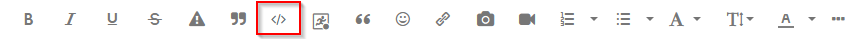## Subroutine error

Hello

I am using my previous code which is working fine, just changed the density. I keep getting warning messages "WARNING: Invalid argument resulted in missing value result."

I check the line that caused this issue and printed it to see all values, but I found no problem with it.

Thank you

``````proc iml;

*seed=0;  NN=10;

theta1=2;   theta2=10;   del=3;

case=2; n1=30; m1=15;   k=J(m1,1,0); K[m1]=n1-m1;       L=K;    	n2=n1;     m2=m1;

**********************************************************************************************************************;

***********************  		Exact OVL 		*******************************;

R=(theta1/theta2);
R_star=((m2-1)/m2)*R;

R1=R_star;

rho_Exact=2*sqrt(R1)/(1+R1);
lambda_Exact=(2*sqrt(R1)/(1+R1))**2;
delta_Exact=1-R1**(1/(1-R1))*abs(1-1/R1);

******************************************************************************;
start Uniform_p(m,R)  global(seed);

V=J(m,1,0); U=J(m,1,0);W=J(m,1);

W=randfun(m,"Uniform");

Do i=1 to m;
Sum=0;
Do j=m-i+1 to m;
Sum=Sum+R[j];
End;
Sum=Sum+i;
V[i]=W[i]**(1/Sum);

End;

Do i=1 to m;
U[i]=1-prod ( V[m: (m-i+1)]   );
End;

Call sort (U);
return U;
Finish;

********************************************************************;

U=uniform_p(m,R);

Y=( log ( 1 -  (1- U)##(1/theta)  ) ) ;

X = - y ##(-1/del);

T=X[m]+2;

Do idx=1 to m-1;
If (( X[idx] < T) & (T <= X[idx+1] )) then j=idx;
End;

If X[m]>T then

Do;
W=Uniform_p(m-j,R);

WE= (1 - Exp(-1/T##del));

X_m_j =( -1/ ( log ( 1 -  ( (1- W)##(1/theta) ) # WE ) ) )##del;

Rm=n-m-sum(R[1:J]);
R2=J(m-j,1,0);R2[m-J]=Rm;
newR=R[1:j]//R2;

End;

Else

Do;
j= m;
newR=R;
End;

return P1;
Finish;

********** Subroutine to compute the MLEs  **************;
*********************************************************;

start MLE(X,n,m,R,J);

Part=1-Exp(- 1/X##(-del));

deno = R[m]*log(part[m])+ sum (log(part)) + sum( (R#log(part))[1:J] ) ;

Theta_MLE = (- m/deno);

return(Theta_MLE);
finish;

******************************************************************************************;

MSE1=J(NN,1,0);MSE2=J(NN,1,0);MSE3=J(NN,1,0);

Do JJ=1 to NN;

*********************************************************************;

K=newK;

L=newL;

End;

** The estimated values of the OVL **;

****** Asymptotic Variance of the Overlap ********;

******   Asymptotic Bias of the Overlap   ********;

Do JJ=1 to NN;

End;

** I used MSE below b/c it provides smaller MSE values;

MSE1 = ( rho_star_Adp    - rho_Exact )##2;
MSE2 = ( lambda_star_Adp - lambda_exact)##2;
MSE3 = ( delta_star_Adp  - delta_exact)##2;

**** Interval estimation using Asymptotic technique ****;

Do JJ=1 to NN;

***************************		 		OVL1			*****************************;

*************************************************************************************;

***************************		 		OVL2			*****************************;

**************************************************************************************;

***************************		 		OVL3			******************************;

end;

***************** 						END Of Simulations	 					************************;
********************************************************************************************************;

MSE11=MSE1[:];
MSE22=MSE2[:];
MSE33=MSE3[:];

****formatting the output *******;

Bias=J(3,1);Length=J(3,1);coverage=J(3,1); MSE=J(3,1,0);

MSE[1,1]= MSE11; 	        MSE[2,1]= (MSE22 );	    MSE[3,1]= (MSE33 );

names={rho,lambda,delta};

Print "Results from T3";
print case  n1 m1;
print names  bias  "    "   MSE   "    " Length "    "   coverage  ;

quit;

``````

1 ACCEPTED SOLUTION

Accepted Solutions

## Re: Subroutine error

I am thinking of adding an if statement "If Y<=0 then Y=0.0001" OR "If y<=0 then Go to Step 1"

Because Y is a vector, some elements can be negative whereas others can be positive. Therefore, you must be careful. An IF-THEN statement checks whether ALL elements of vector satisfy the logical condition. See IF-THEN logic with matrix expressions - The DO Loop (sas.com)

It is always better to understand WHY a situation is occurring rather than try to patch it up after it has occurred. I don't know what distribution you are trying to simulate, but I can tell you that the express

y = log ( 1 - (1- U)##(1/theta) ) ;

is negative when U is in the interval (0,1) and theta > 0.

5 REPLIES 5

## Re: Subroutine error

Show us the entire LOG for this PROC IML so we can see where the error appeared. Please copy the log as text and paste it into the window that appears when you click on the </> icon.--
Paige Miller

## Re: Subroutine error

Looking at the log is essential to understanding errors. In this case, the log tells you

``````WARNING: Invalid argument resulted in missing value result.

count     : number of occurrences is 15
operation : ## at line 72 column 1
operands  : y, _TEM1002
Y     15 rows      1 col     (numeric)

_TEM1002      1 row       1 col     (numeric)

-0.333333

statement : ASSIGN at line 72 column 1
traceback : module ADAPTIVE at line 72 column 1
``````

and Line 72 is the statement

``72 X = - y ##(-1/del);``

The _TEM1002 variable is the temporary expression in parentheses, so apparently
(-1/del) = -0.3333
Apparently, 15 values in the Y vector are nonpositive. The expression
y##(-1/del)
is undefined when y < = 0 and the power is a floating point number.

If DEL is an integer, you can rewrite the expression as
X = -1/ x##del;

which should be valid for y ^= 0.

## Re: Subroutine error

Thank you all for your reply, "del" is not an integer (It could but not necessary).

I am thinking of adding an if statement "If Y<=0 then Y=0.0001" OR "If y<=0 then Go to Step 1" were "Step 1" is defined to take me back to  the beginning of the simulation. However, I tried to change the seed and everything is working fine.  Not sure if I am fooling myself or not. Please advise if any of these ideas are legitimate.

Thank you

## Re: Subroutine error

I am thinking of adding an if statement "If Y<=0 then Y=0.0001" OR "If y<=0 then Go to Step 1"

Because Y is a vector, some elements can be negative whereas others can be positive. Therefore, you must be careful. An IF-THEN statement checks whether ALL elements of vector satisfy the logical condition. See IF-THEN logic with matrix expressions - The DO Loop (sas.com)

It is always better to understand WHY a situation is occurring rather than try to patch it up after it has occurred. I don't know what distribution you are trying to simulate, but I can tell you that the express

y = log ( 1 - (1- U)##(1/theta) ) ;

is negative when U is in the interval (0,1) and theta > 0.

## Re: Subroutine error

Thank you for your suggestions and help.

I believe I know the reason behind it.  It depends on what data is simulated some of the values of Y are extremely small, not many but even one value can cause a damage!

I noticed that for some seed values I may get no outliers

From The DO Loop